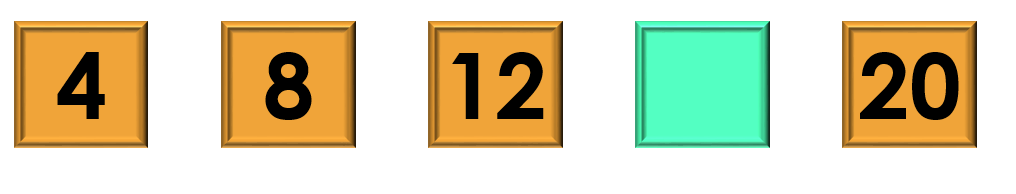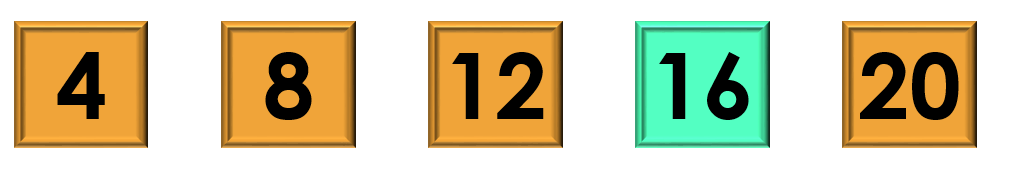1
visibility

Consider the given number pattern and identify the missing number.• A

12

• B

14

• C

16

• D

18

The correct answer is 16.

Solution:

Considering the given number pattern.Here, the numbers are increasing.
So, to find the missing number, we first find the rule.

Note that:
4 + 4 = 8
8 + 4 = 12
12 + 4 = 16
16 + 4 = 20

So, the rule is "add 4".Then, the missing number is 16.